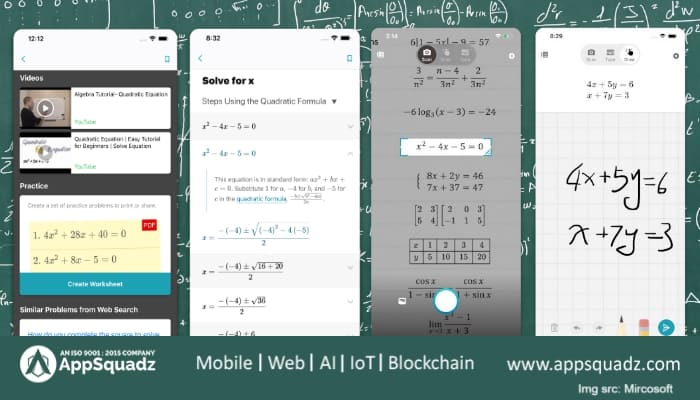# Microsoft’s Math Solver App is out, applies AI to solve Mathematical ProblemsMicrosoft's Math Solver App

Microsoft has launched the Math Solver app, which is an app-in-one solution to solve mathematical problems instantly for Android or iOS devices. The app runs by applying artificial intelligence (AI) which identifies numerical questions and suggests solutions for an extensive array of mathematical concepts. The users of the Microsoft Math Solver app need to add their mathematical questions by drawing, scanning, inserting pictures or typing on the screen. Additionally, the app is composed in a way that presents graphs and a step-by-step explanation of the answers.

## How it works-

In the case of beginning with the Microsoft Math Solver App, you either have to capture a picture of your math problem or type the same through your built-in scientific calculator on the screen. Likewise, you can even add your problem statements by drawing the pictures using your fingers or stylus on the smartphone’s screen or tablet’s screen. After your question instructions are inserted, the app will make use of its AI algorithms to identify your instructions given and afterward show the question on your smartphone’s screen.

Once the AI algorithms identify and understand your mathematical queries, the Microsoft Math Solver app presents you with instant explanations to your questions asked. Not only instant solutions but the app also provides highlights step-by-step explanation to the questions so that you learn to solve your mathematical questions without taking the help of the app.

### Which Languages it Support-

In the Microsoft Math Solver app, Microsoft has presented language support for 22 languages. Among the 22 languages, 12 Indian languages are included which are namely Assamese, Bengali, Gujarati, Hindi, Kannada, Marathi, Punjabi, and Tamil, to name a few. Foreign languages like German, Spanish, and Russian are also included in the language support. The language support is beneficial for the users as they can customize as per their preferred language to understand how different math concepts function with different questions.

### How to Use it-

The Microsoft Math Solver app offers added learning support, which covers video tutorials and related worksheets, making it apparent for the students to practice and understand different concepts applied in the mathematical problems. Microsoft Math Solver provides multiple approaches to solve a single mathematical problem so that students can answer questions without finding any glitch. To make users understand the correlations efficiently, Microsoft has incorporated an interactive graph to the app. Thus, for linear and non-linear functions, you may scan or type and plot X-Y data tables.

Furthermore, using Bing the Microsoft Math Solver app notes related questions from Web search. Besides this, you also get similar concepts based on your mathematical problems. You have the option to bookmark questions of your choice or view the history of the app so that you can find the details of the math’s questions whenever needed. When you are on the app, you will also find the list of some examples for the sake of practicing the concepts.

You will find concepts such as algebra, fractions, rational equations, quadratic equations, arithmetic, trigonometry, matrix multiplication, and differentiation, and many more. The Microsoft Math Solver app is a distinct offering for the students who are weak at mathematics. This handy app comes certainly with simple problem-solving capability. The way the Artificial Intelligence works in this app makes it the all-inclusive solution for the students.

### Mathematical concepts supported on Microsoft Math Solver app:

Elementary: Arithmetic, real & complex numbers, LCM, GCD, factors, roman numerals

Pre-Algebra: Radicals and exponents, fractions, matrices, determinants

Algebra: Quadratic equations, inequalities, rational expressions, linear equations, quadratic and exponential graphs, word problems on math concepts, number theory, probability, volume, surface & area

Basic Calculus: Summations, Limits, derivatives, integrals

Statistics: Mean, Median & Mode, Standard Deviation, permutations & combinations

The Android users and iOS users can seek help in solving your math problems on the Microsoft Math Solver app by downloading its package from the Google Play Store and Apple App Store, respectively. For compatibility, make sure your device is running at least on Android 5.0 Lollipop or iOS 11.0 to be compatible with the app.